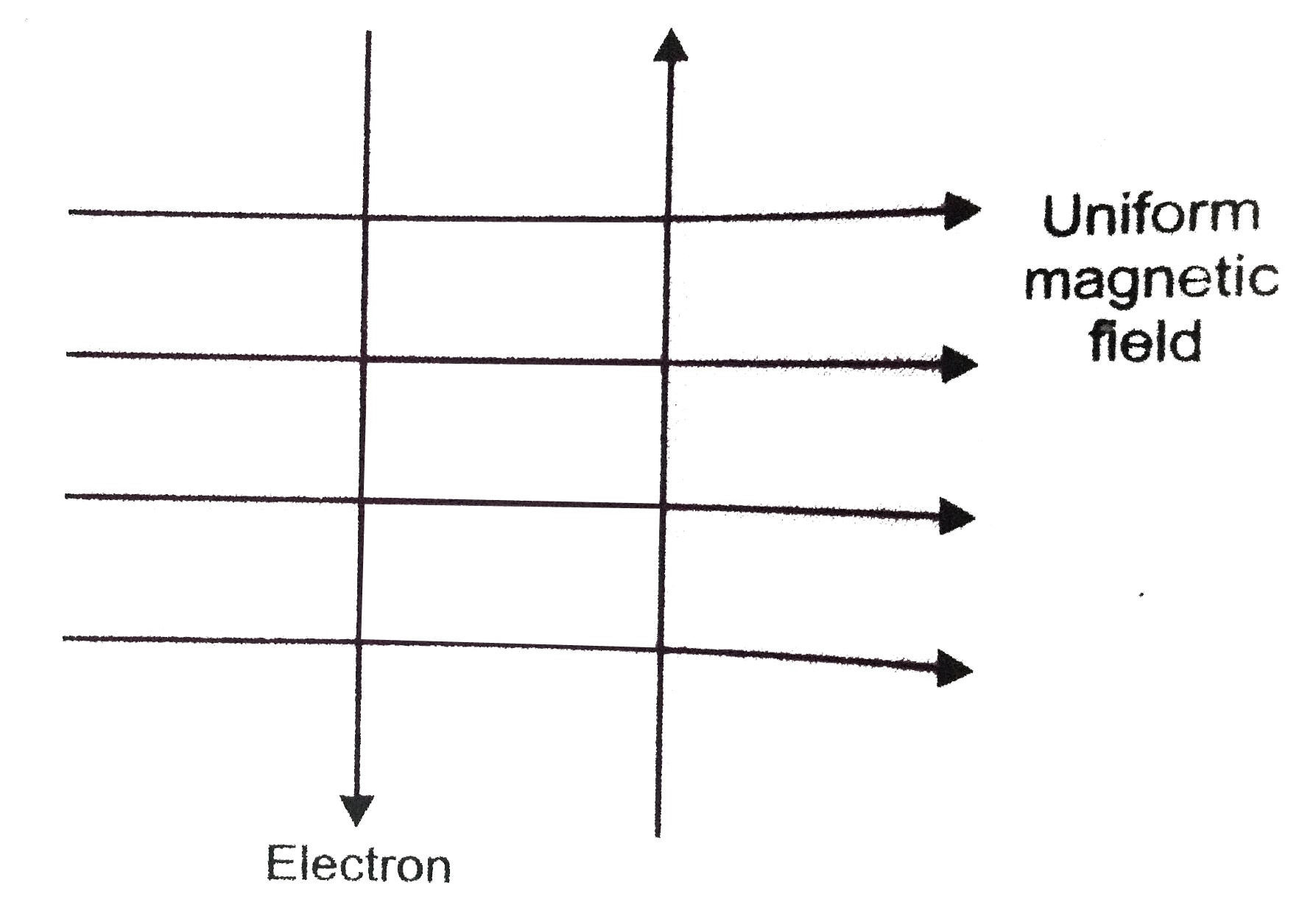# A uniform magnetic field exists in the plane of paper pointing from left to right as shown in figure. In the field, an electron and a proton move as s

74 views
in Physics
A uniform magnetic field exists in the plane of paper pointing from left to right as shown in figure. In the field, an electron and a proton move as shown. The electron and the proton experience:A. (a) forces both pointing into the plane of paper
B. (b) forces both pointing out of the plane of paper
C. (c) forces poiting into the plane of paper and out of the plane of paper, respectively.
D. (d) forces pointing opposite and along the direction of the uniform magnetic field respectively

by (37.4k points)